# Chapter 8 Section 1 Describing Chemical Reactions A

• Slides: 18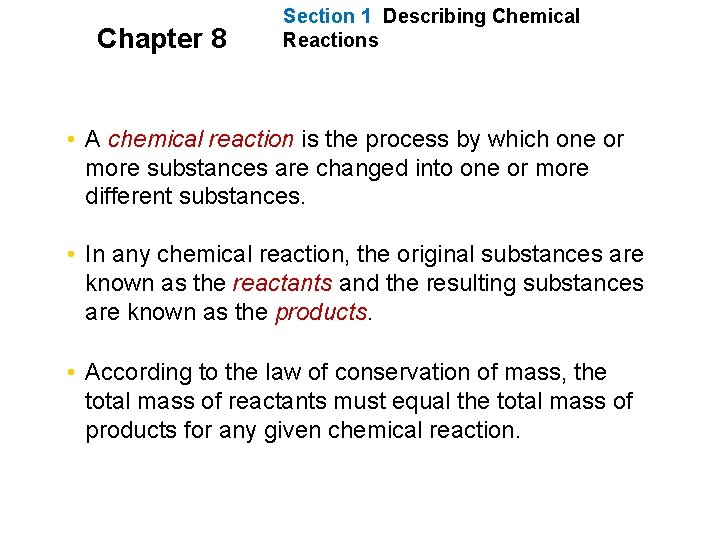Chapter 8 Section 1 Describing Chemical Reactions • A chemical reaction is the process by which one or more substances are changed into one or more different substances. • In any chemical reaction, the original substances are known as the reactants and the resulting substances are known as the products. • According to the law of conservation of mass, the total mass of reactants must equal the total mass of products for any given chemical reaction.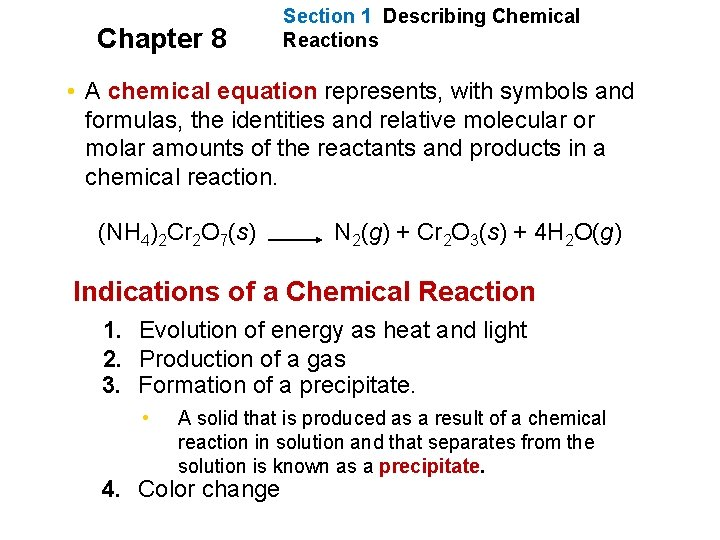Chapter 8 Section 1 Describing Chemical Reactions • A chemical equation represents, with symbols and formulas, the identities and relative molecular or molar amounts of the reactants and products in a chemical reaction. (NH 4)2 Cr 2 O 7(s) N 2(g) + Cr 2 O 3(s) + 4 H 2 O(g) Indications of a Chemical Reaction 1. Evolution of energy as heat and light 2. Production of a gas 3. Formation of a precipitate. • A solid that is produced as a result of a chemical reaction in solution and that separates from the solution is known as a precipitate. 4. Color changeChapter 8 Section 1 Describing Chemical Reactions Characteristics of Chemical Equations, Word and Formula Equations • A word equation is an equation in which the reactants and products in a chemical reaction are represented by words. • A word equation is qualitative • example: methane + oxygen carbon dioxide + water • A formula equation represents the reactants and products of a chemical reaction by their symbols or formulas. • example: The formula equation for the reaction of methane and oxygen is CH 4(g) + O 2(g) CO 2(g) + H 2 O(g) (not balanced)Chapter 8 Section 1 Describing Chemical Reactions Symbols Used in Chemical Equations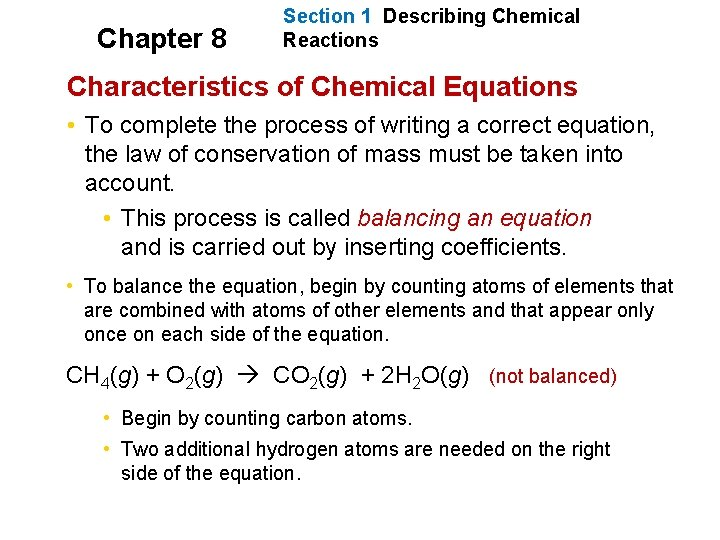Chapter 8 Section 1 Describing Chemical Reactions Characteristics of Chemical Equations • To complete the process of writing a correct equation, the law of conservation of mass must be taken into account. • This process is called balancing an equation and is carried out by inserting coefficients. • To balance the equation, begin by counting atoms of elements that are combined with atoms of other elements and that appear only once on each side of the equation. CH 4(g) + O 2(g) CO 2(g) + 2 H 2 O(g) (not balanced) • Begin by counting carbon atoms. • Two additional hydrogen atoms are needed on the right side of the equation.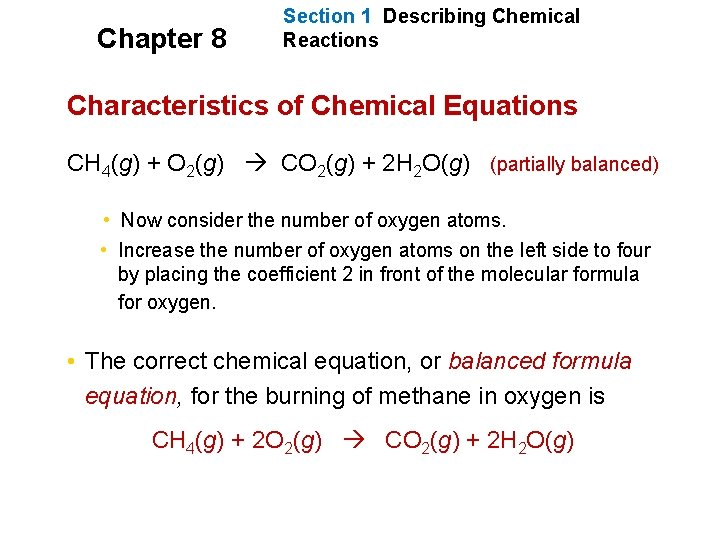Chapter 8 Section 1 Describing Chemical Reactions Characteristics of Chemical Equations CH 4(g) + O 2(g) CO 2(g) + 2 H 2 O(g) (partially balanced) • Now consider the number of oxygen atoms. • Increase the number of oxygen atoms on the left side to four by placing the coefficient 2 in front of the molecular formula for oxygen. • The correct chemical equation, or balanced formula equation, for the burning of methane in oxygen is CH 4(g) + 2 O 2(g) CO 2(g) + 2 H 2 O(g)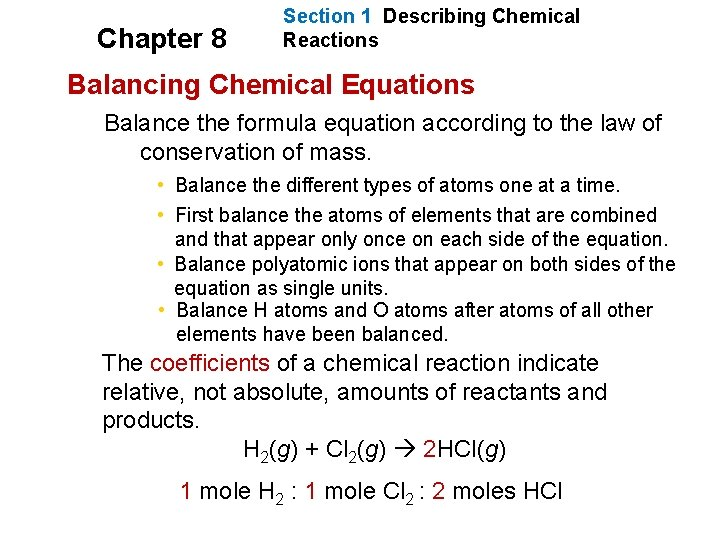Chapter 8 Section 1 Describing Chemical Reactions Balancing Chemical Equations Balance the formula equation according to the law of conservation of mass. • Balance the different types of atoms one at a time. • First balance the atoms of elements that are combined and that appear only once on each side of the equation. • Balance polyatomic ions that appear on both sides of the equation as single units. • Balance H atoms and O atoms after atoms of all other elements have been balanced. The coefficients of a chemical reaction indicate relative, not absolute, amounts of reactants and products. H 2(g) + Cl 2(g) 2 HCl(g) 1 mole H 2 : 1 mole Cl 2 : 2 moles HCl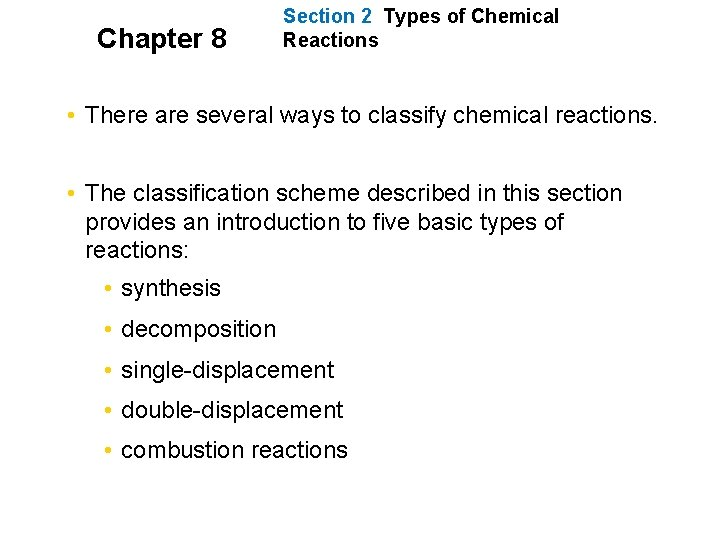Chapter 8 Section 2 Types of Chemical Reactions • There are several ways to classify chemical reactions. • The classification scheme described in this section provides an introduction to five basic types of reactions: • synthesis • decomposition • single-displacement • double-displacement • combustion reactions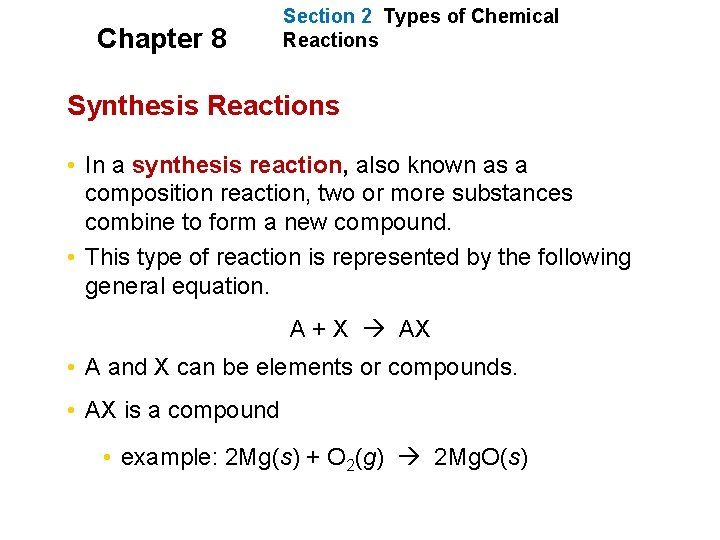Chapter 8 Section 2 Types of Chemical Reactions Synthesis Reactions • In a synthesis reaction, also known as a composition reaction, two or more substances combine to form a new compound. • This type of reaction is represented by the following general equation. A + X AX • A and X can be elements or compounds. • AX is a compound • example: 2 Mg(s) + O 2(g) 2 Mg. O(s)Chapter 8 Section 2 Types of Chemical Reactions Decomposition Reactions • In a decomposition reaction, a single compound undergoes a reaction that produces two or more simpler substances. s or compounds. • Decomposition reactions are the opposite of synthesis reactions. • They are represented by the following general equation. AX A + X • AX is a compound. • A and X can be elements or compounds. • example:Chapter 8 Section 2 Types of Chemical Reactions Decomposition Reactions, continued Decomposition of Binary Compounds • The decomposition of a substance by an electric current is called electrolysis. • example: • Oxides of the less-active metals, which are located in the lower center of the periodic table, decompose into their elements when heated. • example:Chapter 8 Section 2 Types of Chemical Reactions Single-Displacement Reactions • In a single-displacement reaction, also known as a replacement reaction, one element replaces a similar element in a compound. • Many single-displacement reactions take place in aqueous solution. • Single-displacement reactions can be represented by the following general equations. A + BX AX + B or Y + BX BY + X • A, B, X, and Y are elements. AX, BX, and BY are compounds. 2 Al(s) + 3 Pb(NO 3)2(aq) 3 Pb(s) + 2 Al(NO 3)3(aq)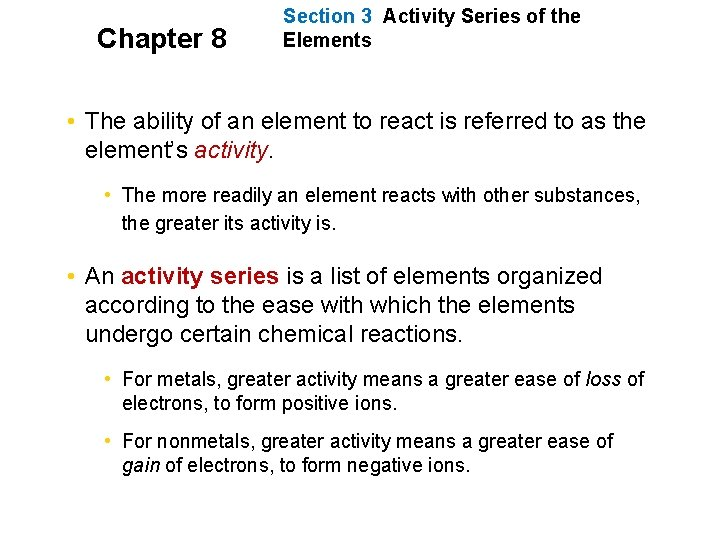Chapter 8 Section 3 Activity Series of the Elements • The ability of an element to react is referred to as the element’s activity. • The more readily an element reacts with other substances, the greater its activity is. • An activity series is a list of elements organized according to the ease with which the elements undergo certain chemical reactions. • For metals, greater activity means a greater ease of loss of electrons, to form positive ions. • For nonmetals, greater activity means a greater ease of gain of electrons, to form negative ions.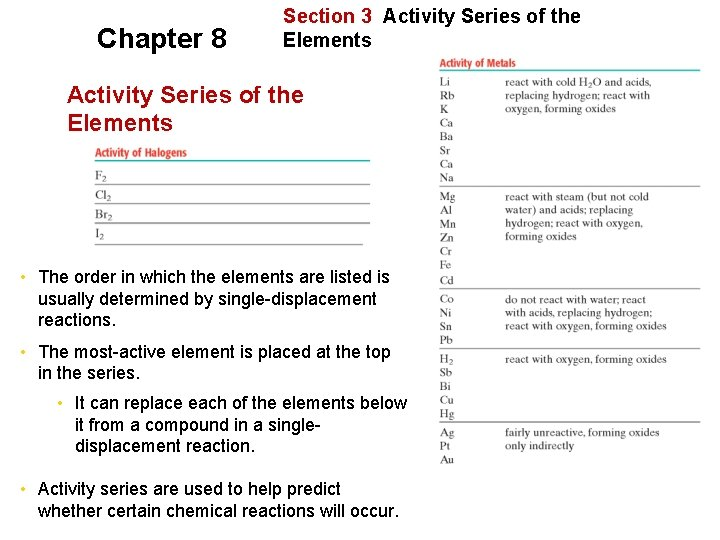Chapter 8 Section 3 Activity Series of the Elements • The order in which the elements are listed is usually determined by single-displacement reactions. • The most-active element is placed at the top in the series. • It can replace each of the elements below it from a compound in a singledisplacement reaction. • Activity series are used to help predict whether certain chemical reactions will occur.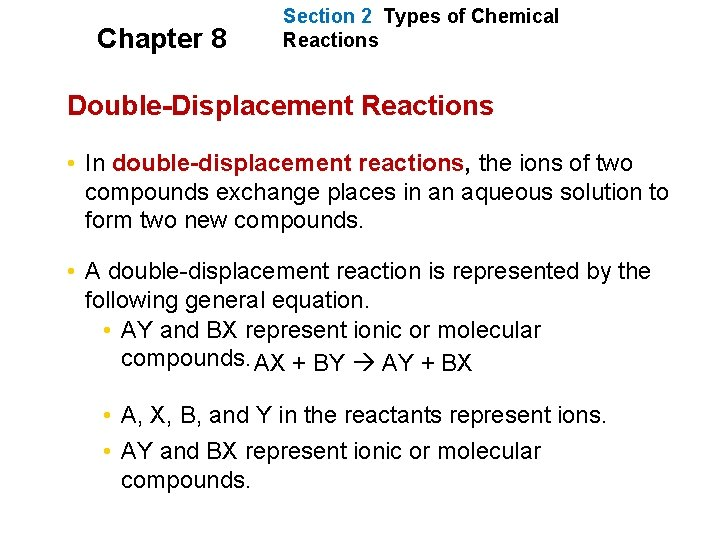Chapter 8 Section 2 Types of Chemical Reactions Double-Displacement Reactions • In double-displacement reactions, the ions of two compounds exchange places in an aqueous solution to form two new compounds. • A double-displacement reaction is represented by the following general equation. • AY and BX represent ionic or molecular compounds. AX + BY AY + BX • A, X, B, and Y in the reactants represent ions. • AY and BX represent ionic or molecular compounds.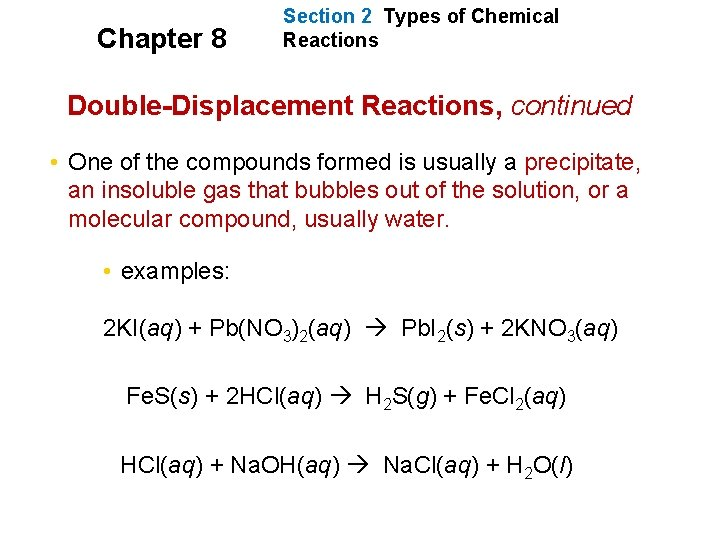Chapter 8 Section 2 Types of Chemical Reactions Double-Displacement Reactions, continued • One of the compounds formed is usually a precipitate, an insoluble gas that bubbles out of the solution, or a molecular compound, usually water. • examples: 2 KI(aq) + Pb(NO 3)2(aq) Pb. I 2(s) + 2 KNO 3(aq) Fe. S(s) + 2 HCl(aq) H 2 S(g) + Fe. Cl 2(aq) HCl(aq) + Na. OH(aq) Na. Cl(aq) + H 2 O(l)Chapter 8 Section 2 Types of Chemical Reactions Combustion Reactions • In a combustion reaction, a substance combines with oxygen, releasing a large amount of energy in the form of light and heat. • example: combustion of hydrogen 2 H 2(g) + O 2(g) 2 H 2 O(g) • example: combustion of propane C 3 H 8(g) + 5 O 2(g) 3 CO 2(g) + 4 H 2 O(g)Chapter 8 Section 2 Types of Chemical Reactions Determining Reaction Types